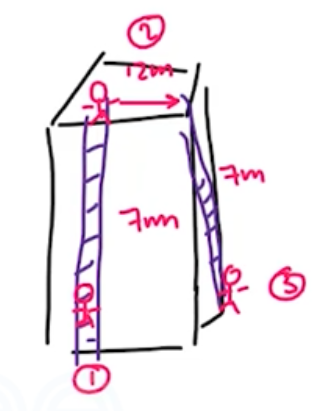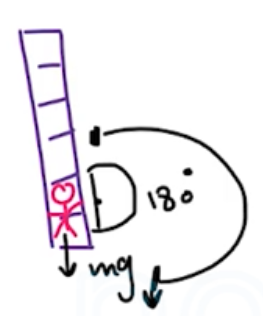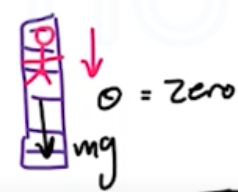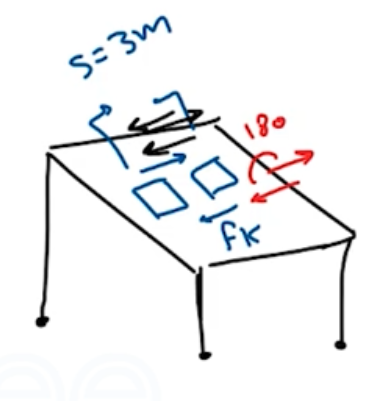Need Help?

Subscribe to Physics 1

###### \${selected_topic_name}
• Notes

$\begin{array}{l}{\text { A } 75-\mathrm{kg} \text { roofer climbs a vertical } 7.0 \text { -m ladder to the flat }} \\ {\text { roof of a house. He then walks } 12 \mathrm{m} \text { on the roof, climbs down }} \\ {\text { another vertical } 7.0 \text { -m lader, and finally walks on the ground back }} \\ {\text { to his starting point. How much work is done on him by gravity }} \\ {\text { (a) as he climbs up; (b) as he climbs down; (c) as he walks on the }}\end{array}$

$\begin{array}{l}{\text { roof and on the ground? (d) What is the total work done on him by }} \\ {\text { gravity during this round trip? (e) On the basis of your answer to }} \\ {\text { part (d), would you say that gravity is a conservative or nonconser- }} \\ {\text { vative force? Explain. }}\end{array}$$w_{g}=m g \cos \theta$

$=75*9.8 \cos 180$

$=-5100J$$w_g=m g \cos \theta$

$=75 *9.8 \cos0$

$=5100\mathrm{J}$$\theta=90^{\circ}$

$w _g=Z e r o$

$w_{total}=w_{g_{1}}+w_{g_{2}}+w_{g_{3}}$

$-5100+0+5100=Zero$

$\begin{array}{l}{\text { A } 0.60-\mathrm{kg} \text { book slides on "horizontal table. The kinetic fric- }} \\ {\text { tion force on the book has magnitude } 1.2 \mathrm{N} \text { . (a) How much work is }} \\ {\text { done on the book by friction during a displacement of } 3.0 \mathrm{m} \text { to the }} \\ {\text { left? (b) The book now slides } 3.0 \mathrm{m} \text { to the right, returning to its }} \\ {\text { starting point. During this second } 3.0-\mathrm{m} \text { displacement, how much }}\end{array}$

$\begin{array}{l}{\text { work is done on the book by friction? (c) What is the total work }} \\ {\text { done on the book by friction during the complete round trip? (d) On }} \\ {\text { the basis of your answer to part (c), would you say that the friction }} \\ {\text { force is conservative or nonconservative? Explain. }}\end{array}$$m=0.6 \mathrm{kg}$

$S=3 m$

$f_{k}=1.2 \mathrm{N}$

$\theta=180^{\circ}$

$W{f_{k}}=f_{k} S \cos \theta$

${=-(1.2)(0.3) \cos 180=-3.6 \mathrm{J}}$

$w_{2} f_{k}=-3.6 J$

$w_\text { net }=-3.6+(-3.6)=-7.2 \mathrm{J}$

$\text { force is Not Conservation. }$